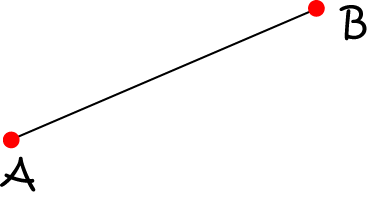# Definition of IntervalAn interval is part of a line that extends between two points.

Some examples of intervals are

• The part of the number line between $2$ and $3$. If this includes the endpoints, we write it using the notation $[2,3]$ (the square brackets mean that the endpoints are included). If it doesn't include the endpoints, we write it using the notation $(2,3)$ (the round brackets mean that the end points aren't included).
• A length of time, marked by fixed starting and finishing times is called a time interval.
• A line segment.

### Description

The aim of this dictionary is to provide definitions to common mathematical terms. Students learn a new math skill every week at school, sometimes just before they start a new skill, if they want to look at what a specific term means, this is where this dictionary will become handy and a go-to guide for a student.

### Audience

Year 1 to Year 12 students

### Learning Objectives

Learn common math terms starting with letter I

Author: Subject Coach
You must be logged in as Student to ask a Question.